# NCERT Solutions for Class 7 Maths Chapter 2 Fractions and Decimals Ex 2.4

NCERT Solutions for Class 7 Maths Chapter 2 Fractions and Decimals Exercise 2.4

Ex 2.4 Class 7 Maths Question 1.
Find:Solution:Ex 2.4 Class 7 Maths Question 2.
Find the reciprocal of each of the following fractions. Classify the reciprocals as proper fractions, improper fractions and whole numbers.(i) Reciprocal of $$\frac{3}{7}=\frac{7}{3}$$, which is improper fraction.
(ii) Reciprocal of $$\frac{5}{8}=\frac{8}{5}$$, which is improper fraction.
(iii) Reciprocal of $$\frac{9}{7}=\frac{7}{9}$$, which is proper fraction.
(iv) Reciprocal of $$\frac{6}{5}=\frac{5}{6}$$, which is proper fraction.
(vi) Reciprocal of $$\frac{12}{7}=\frac{7}{12}$$, which is proper fraction.
(vi) Reciprocal of $$\frac{1}{8}=8$$, which is whole number.
(vii) Reciprocal of $$\frac{1}{11}=11$$, which is whole number.

Ex 2.4 Class 7 Maths Question 3.
Find: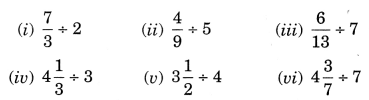Solution: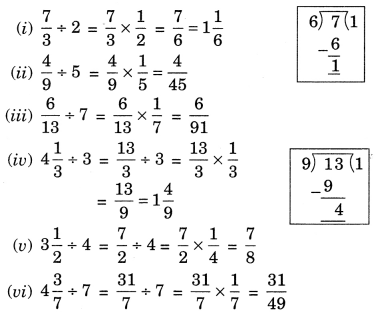Ex 2.4 Class 7 Maths Question 4.
Find: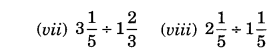Solution: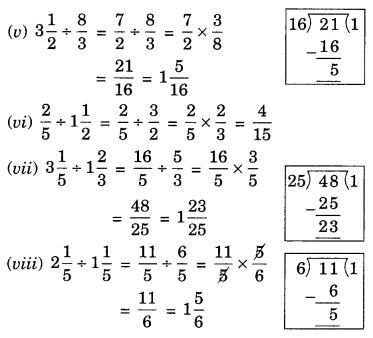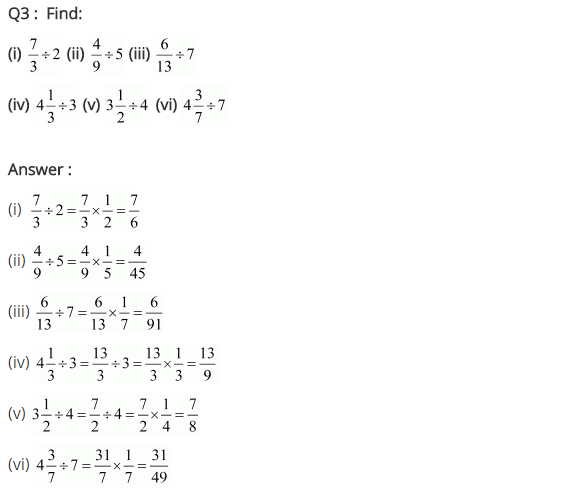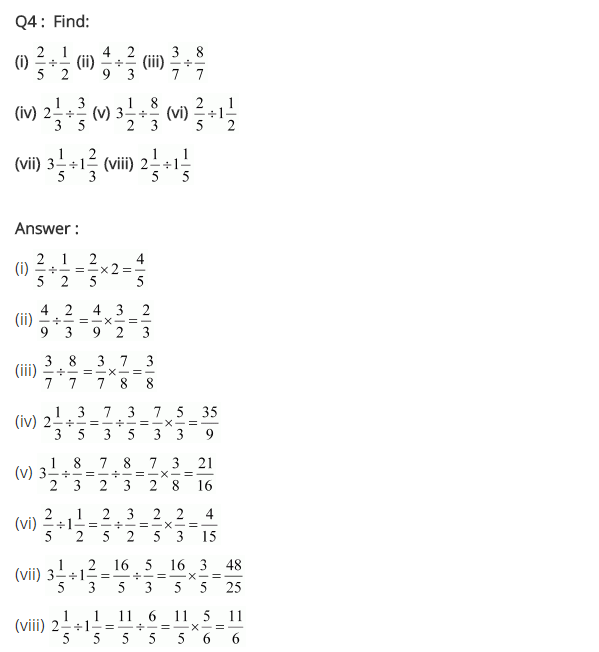+